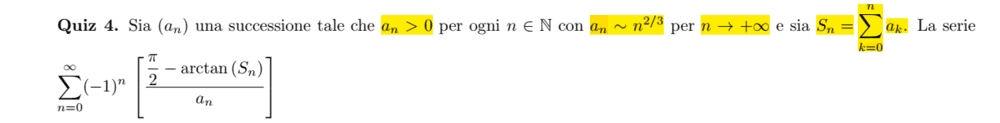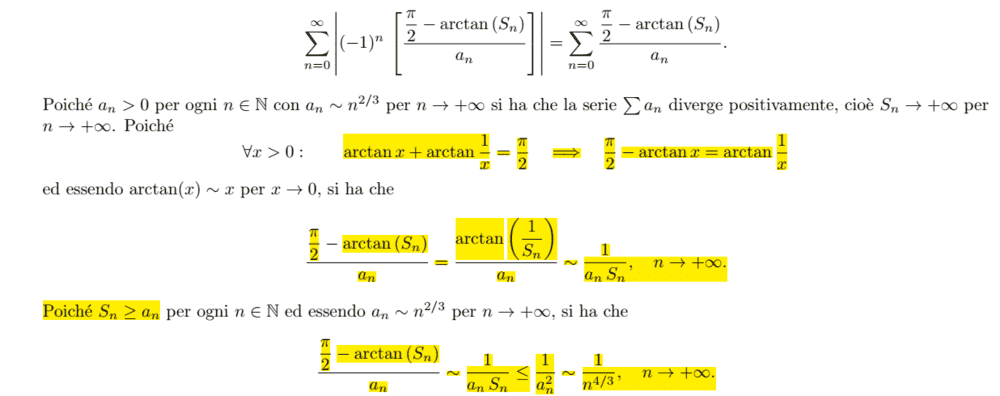# Studying the convergence of a series with an arctangent of a partial sum

Amaelle
Homework Statement:
look at the image below
Relevant Equations:
asymptotic behaviour, limit of partial sum
Greeting
I'm trying to study the convergence of this serieI started studying the absolute convergence
because an≈n^(2/3) we know that Sn will be divergente S=∝ so arcatn (Sn)≤π/2 and the denominator would be a positive number less than π/2, and because an≈n^(2/3) and we know 1/n^(2/3) > 1/n so we conclude that this serie divergent (not absolutely convergent till this point)

but here is the solution of the bookso according to them the serie is absolutely convergent

I would be grateful if someone could explain me where I get wrong in my reasoning

Best

Gold Member
You don’t seem to investigate smallness of numerator as the answer does.

Amaelle
I consider it as a postive number let's call it A 0<A<pi(2) A/n^2/3 > A/n and we know A/n is divergent so should be A/n^2/3 ?
where is the flaw in my reasoning?

Staff Emeritus
Gold Member
But what is A is really, really, really small?

If A=1/n for example, then A/n does converge.

You assumed there was some constant ##A>0## that was a lower bound for the numerator, but no such constant exists.

•Amaelle
Gold Member
The solution estimates A as 1/Sn < 1/an

•Amaelle
Amaelle
But what is A is really, really, really small?

If A=1/n for example, then A/n does converge.

You assumed there was some constant ##A>0## that was a lower bound for the numerator, but no such constant exists.
thanks alot
I want to ask about the difference between this case and between when we have for a example a dominator with sin(n) and we just say that |sin(n)|<1
what is the difference between this case and the exercice below?

Staff Emeritus
Gold Member
Sure. ##|\sin(n)| < 1## is a true statement.

You want to do something like

##|\pi/2 - \arctan(n) | > 0.01##, where 0.01 is a number I just came up with (any number would work). But that's not a true statement, so you can't use it.

•Amaelle
Amaelle
Sure. ##|\sin(n)| < 1## is a true statement.

You want to do something like

##|\pi/2 - \arctan(n) | > 0.01##, where 0.01 is a number I just came up with (any number would work). But that's not a true statement, so you can't use it.
thanks a million!